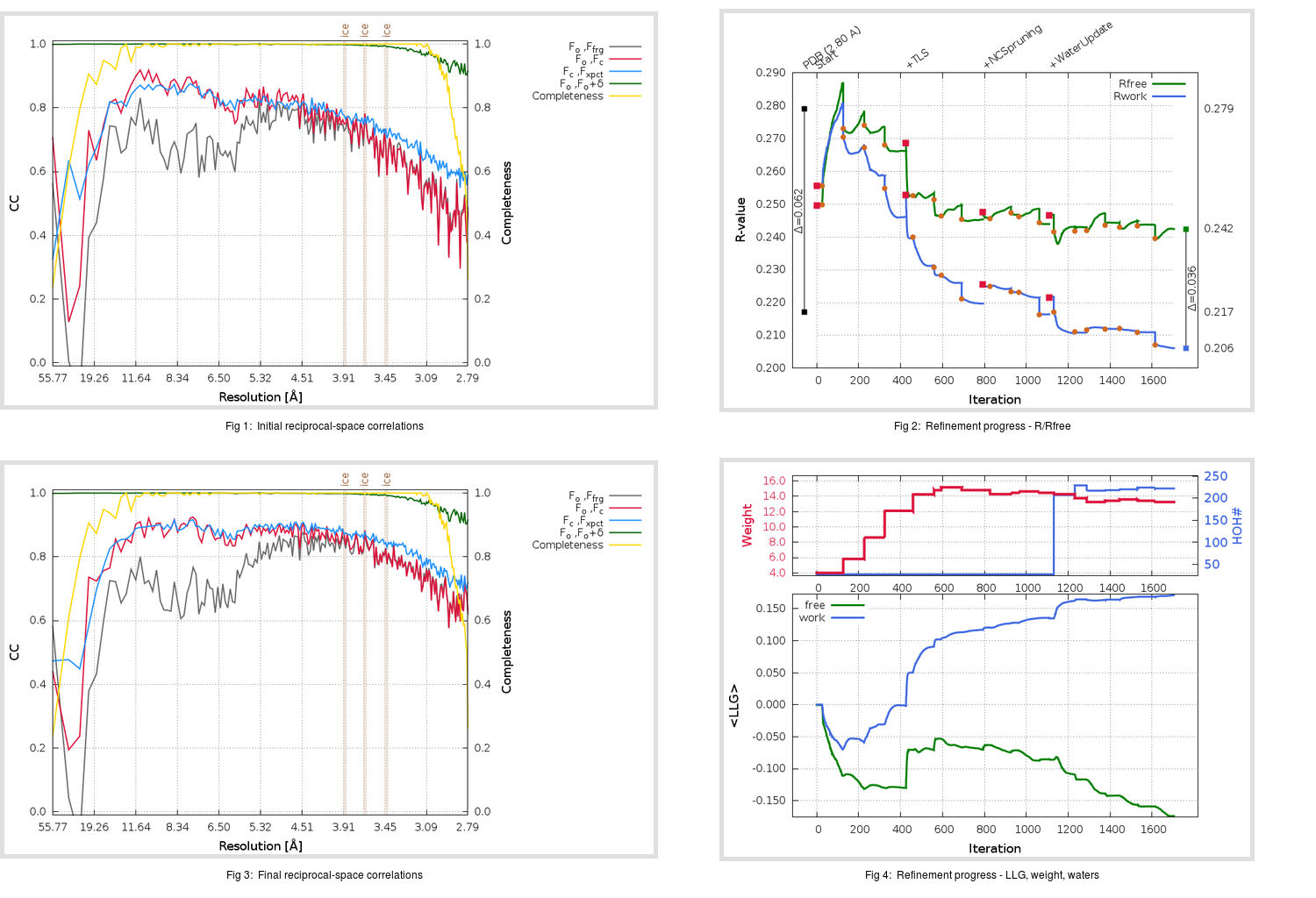Content:

```    Diffraction limits & principal axes of ellipsoid fitted to diffraction cut-off surface:
2.708         0.8170   0.0000  -0.5766       0.672 a* - 0.740 c*
2.832         0.0000   1.0000   0.0000       b*
2.826         0.5766   0.0000   0.8170       0.492 a* + 0.870 c*
```

## Deposited

` `
 Date deposited Date data collection Resolution R, Rfree 20080501 2.80 0.2140 0.2790

Molprobity (CCP4 7.0 version) summary:

```Ramachandran outliers =   1.77 %
favored =  89.33 %
Rotamer outliers      =  11.06 %
C-beta deviations     =     5
Clashscore            =   9.87
RMS(bonds)            =   0.0100
RMS(angles)           =   1.28
MolProbity score      =   2.88
Resolution            =   2.80
R-work                =   0.2140
R-free                =   0.2790
```

```Number of waters      =    27

<B> (all atoms) =   74.50 ( sd =   22.17 ) for      12643 non-hydrogen atoms
<B>   (protein) =   74.35 ( sd =   22.06 ) for      12522 non-hydrogen atoms
<B>     (water) =   51.78 ( sd =    9.95 ) for         27 non-hydrogen atoms
<B>    (others) =  101.16 ( sd =   19.95 ) for         94 non-hydrogen atoms

B min/max       (all non-hydrogen atoms) =   35.80 /  140.75
B min/max   (protein non-hydrogen atoms) =   35.80 /  140.75
B min/max     (water non-hydrogen atoms) =   37.89 /   76.15
B min/max     (other non-hydrogen atoms) =   53.92 /  129.01
```

## BUSTER (re-)refinement

` `

Molprobity (CCP4 7.0 version) summary:

```Ramachandran outliers =   0.92 %
favored =  94.70 %
Rotamer outliers      =  11.50 %
C-beta deviations     =     9
Clashscore            =   5.72
RMS(bonds)            =   0.0123
RMS(angles)           =   1.63
MolProbity score      =   2.48
Resolution            =   2.79
R-work                =   0.2061
R-free                =   0.2424
```

```Number of waters      =   222

<B> (all atoms) =   94.88 ( sd =   27.14 ) for      12838 non-hydrogen atoms
<B>   (protein) =   94.96 ( sd =   26.71 ) for      12522 non-hydrogen atoms
<B>     (water) =   68.93 ( sd =   13.23 ) for        222 non-hydrogen atoms
<B>    (others) =  146.30 ( sd =   30.10 ) for         94 non-hydrogen atoms

B min/max       (all non-hydrogen atoms) =   40.48 /  189.44
B min/max   (protein non-hydrogen atoms) =   44.88 /  181.78
B min/max     (water non-hydrogen atoms) =   40.48 /  118.08
B min/max     (other non-hydrogen atoms) =   68.93 /  189.44
```

Refinement progression:Results:

` `
 File Remark 3D0G_aB_refine.01_04_refine.pdb.gz exact refinement commands are in header 3D0G_aB_refine.01_04_refine.mtz.gz including original deposited data and several re-refinement map coefficients 3D0G_aB_refine.01_04_BUSTER_model.cif.gz including any non-standard compound restraints 3D0G_aB_refine.01_04_BUSTER_refln.cif.gz